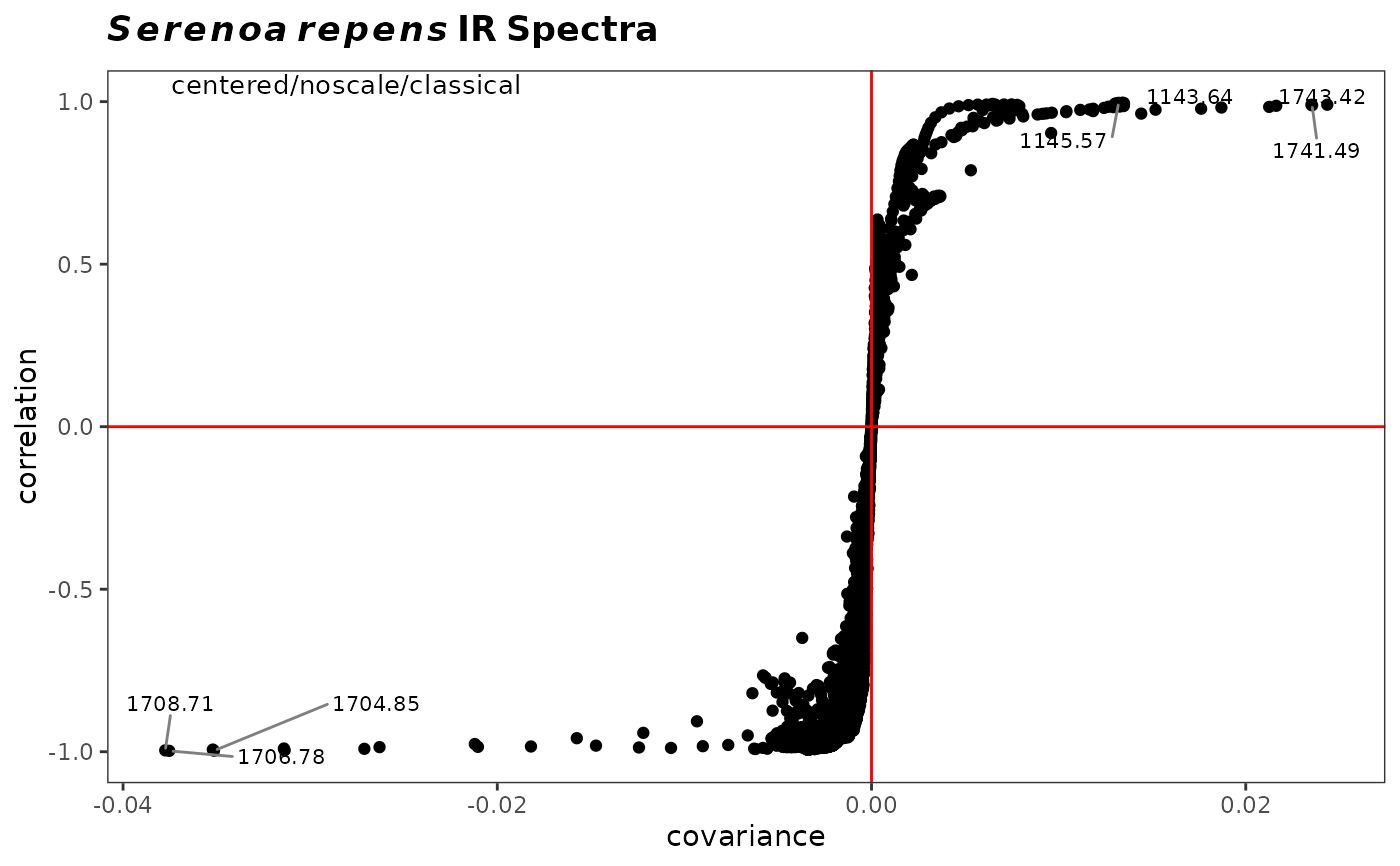Produces a scatter plot of the correlation of the variables against their covariance for a chosen principal component. It allows visual identification of variables driving the separation and thus is a useful adjunct to traditional loading plots.

sPlotSpectra(spectra, pca, pc = 1, tol = 0.05, ...)

## Arguments

spectra

An object of S3 class Spectra().

pca

The result of a pca calculation on Spectra (i.e. the output from c_pcaSpectra or r_pcaSpectra).

pc

An integer specifying the desired pc plot.

tol

A number describing the fraction of points to be labeled. tol = 1.0 labels all the points; tol = 0.05 labels approximately the most extreme 5 percent. Set to 'none' to completely suppress labels. Note that a simple approach based upon quantiles is used, assumes that both x and y are each normally distributed, and treats x and y separately. Thus, this is not a formal treatment of outliers, just a means of labeling points. Groups are lumped together for the computation.

...

Parameters to be passed to the plotting routines. Applies to base graphics only.

## Value

The returned value depends on the graphics option selected (see GraphicsOptions()).

• base: None. Side effect is a plot.

• ggplot2: The plot is displayed, and a ggplot2 object is returned if the value is assigned. The plot can be modified in the usual ggplot2 manner.

## Author

Bryan A. Hanson (DePauw University), Tejasvi Gupta, Matthew J. Keinsley.

## Examples


# This example assumes the graphics output is set to ggplot2 (see ?GraphicsOptions).
library("ggplot2")
data(SrE.IR)
pca <- c_pcaSpectra(SrE.IR)
myt <- expression(bolditalic(Serenoa) ~ bolditalic(repens) ~ bold(IR ~ Spectra))
p <- sPlotSpectra(spectra = SrE.IR, pca = pca, pc = 1, tol = 0.001)
p <- p + ggtitle(myt)
p